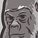# New to Qlik Sense

If you’re new to Qlik Sense, start with this Discussion Board and get up-to-speed quickly.

Announcements
Read about the latest Qlik Community enhancements on the Community News blog!
cancel
Showing results for
Did you mean:Contributor II

## Using a variable containing a function in the element list of a set analysis

Hi All,

I have a straight table (chart) with one dimension as branches of industry.
The expressions count the number of customers in that branche that have used a certain feature from our software in weeknumber X. I have three expressions; where X is the previous week, the current week and the next week.

I used three variables for these weeks in the notation YYYYWW where YYYY is the year (e.g. 2021) and WW is the week number (e.g. 18). So for week 18 of 2021 the outcome is 202118 (a integer number).

The variable for the current week is set up as: Year(Today())*100+Week(Today())
I used the variable name vThisYearWeek.
Evaluating this variable in a text box wityh the statement =\$(vVariableName) results in the correct number 202118.

My expression formula in the table is:
Count(DISTINCT {1<PlanningId={"*"},PlanningStatusId={2,3},YearWeek={"=\$(vThisYearWeek)"}>}OgId)

YearWeek is a field in the data table.

But in stead of resulting in the count for this week, it results in a count as if the YearWeek is not filtered. As if the expression has been: Count(DISTINCT {1<PlanningId={"*"},PlanningStatusId={2,3}>}OgId)

When I subsitute the variable by the constant value 202118, then I get the correct results:
Count(DISTINCT {1<PlanningId={"*"},PlanningStatusId={2,3},YearWeek={202118}>}OgId)
So something in the evaluation for YearWeek makes it invalid or obsolete when the variable is used?

I have search all over the forum and tried different approaches but to no avail.
When using constants the script of my dashboard must be edited every week so that is no option.

I hope someone can help.

Thanks,
Hans

For every new problem someone probably found a solution already ...
Labels (4)

• ### variable

11 RepliesContributor II
Author

Hi Marcus,

There is no comment in the variable. The variable's content is: Year(Today())*100+Week(Today())

And when I evaluate it (for example in the label of the expression column) then I get the correct result.
I really do not get what is going on here. As I have a background as c, c++ and c# programmer, I think that the evaluation in the element list of the set expression results in a different type. That is; not a numeric (integer) value.

Or that it fails to evaluate and uses the formula as comparison.
Because the set analysis results in the comparison evaluating to false.

I also have not seen another example where a function present in a variable is used in the element list of a set analysis. Maybe it just is not made to work.

-Hans

For every new problem someone probably found a solution already ...MVP & Luminary

By the use of variables it's an important to consider how the variable is created and where and how they is then called. If a variable starts with an equal (=) sign it is equivalent to LET within the script and means that the right part of the variable statement is evaluated at once and this result is assigned to the variable. If there is no equal sign it is equivalent to SET and means the right part is taken as string.

= year(today()) * 100 + week(today()) /* 1. */

results in 202120 and

year(today()) * 100 + week(today()) /* 2. */

results in year(today()) * 100 + week(today())

Of course this makes a difference when the variable is called. In the first case you may call it like:

sum({< YearWeek = {\$(var)}>} Value)

and in the second case like:

sum({< YearWeek = {"\$(=var)"}>} Value)

Further important is that in this case a string-comparing happens and both sides needs to have the same format. Would you intend to call not single values else a range of them, for example by:

sum({< YearWeek = {"<=\$(=var)"}>} Value)

the use of <= forced a numeric interpretation fully independent of the formatting - of course both sides needs to have a numeric value-part for it.

Another not the variable and/or set analysis related point is the use of today() which will return the today-value from the opening-time of the application - if you don't close the app in the evening and looking on the app on the next day the today-value isn't current anymore. But you could change this behaviour with an appropriate parameter, maybe this one: today(0).

Beside this it's further essentially to consider that Qlik is case sensitive by tables/fields/variable-names - the smallest differences will lead to a mismatch/error. Therefore I suggest that you check this again especially if there are probably multiple very similar fields and/or variables that you always used the right ones.

- MarcusTags
Community Browser